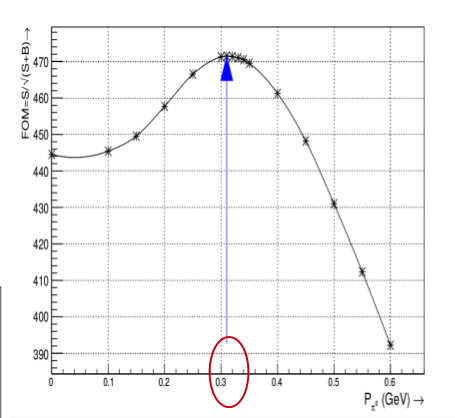# How to draw a graph for optimization using TGraph?

Dear Experts,
I am very much new to this type of problems. I want to do optimisation of one variable(pi0mom1) i.e. I want to plot F.O.M along y-axis and that variable along x-axis. F.O.M = Signal / [Signal + Background]. My condition for signal is that dstrf==1 and for background it is dstrf==5. I tried to write a code for it. Please have a look at it. But I am not getting the plot the way I wanted. Could you please correct that portion of the code or suggest me what to do.

``````void opti()
{

TChain chain1("h1");

Float_t pi0mass1, dstrf,pi0mass2,pi0mom1,pi0mom2,d0mass,deltam,mass,mom ;

Float_t sc=1;//760318/249999; //761202 for mm amd 760318 for ee

Int_t i,j;
int n1=50;
Float_t h1=0.02 ;
Float_t S1,B1,x_var,Fom1,S2,B2 ;
Float_t sig1,sig2,bkg1,bkg2,x1,x2,FOM1=-200,FOM2=-200,LEcut,REcut;

for (i=0; i<n1; i++){
x_var[i] = 8.5+i*h1;
sig1=bkg1=0;
for (j=0; j<chain1.GetEntries(); j++){
chain1.GetEntry(j);

if(pi0mom1>x_var[i] && pi0mom1<6 && d0mass>1.78 && d0mass<1.95 && dstrf==1){
++sig1; }}
S1[i] = sig1/500000.0;
}

for (j=0; j<chain1.GetEntries(); j++){
chain1.GetEntry(j);
if(pi0mom1>x_var[i] && pi0mom1<6 && d0mass>1.78 && d0mass<1.95 && dstrf==5){
++bkg1;
}
}

Fom1[i]= S1[i]/ S1[i] + B1[i];
dF1[i] = sqrt((sig1*.000002*.000002)/(B1[i]*B1[i])+(bkg1*S1[i]*S1[i])/(4*B1[i]*B1[i]*B1[i]*B1[i]*bkg1));
dx[i]=0;
if(Fom1[i]>FOM1){
LEcut=x_var[i];
FOM1=Fom1[i];}

std::cout<<x_var[i]<<std::endl;

TCanvas *c1 = new TCanvas("c1","",0,0,700,400);
c1->SetFrameFillColor(kWhite);
c1->SetFillColor(kWhite);
c1->Divide(2,1);

TGraph *gr1 = new TGraphErrors(n1,x_var,Fom1);
c1->cd(1);
gr1->SetMarkerStyle(21);
gr1->Draw("AC");

}
``````

Regards

ROOT Version: Not Provided
Platform: Not Provided
Compiler: Not Provided

Can you post here the plot you get and tell what’s wrong with it ? (we cannot run your macro, data files are needed)

Thank you very much for the response. I want following kind of graph.``````gr1->Draw("AC*");
``````

Thanks for the response. The array I defined to calculate FOM. I think it is not calculating that properly. Could you please check that part ?

We cannot run your macro to see what could be wrong: the data are missing.

When you reach these lines

``````   Fom1[i]= S1[i]/ S1[i] + B1[i];
dF1[i] = sqrt((sig1*.000002*.000002)/(B1[i]*B1[i])+(bkg1*S1[i]*S1[i])/(4*B1[i]*B1[i]*B1[i]*B1[i]*bkg1));
dx[i]=0;
if(Fom1[i]>FOM1){
LEcut=x_var[i];
FOM1=Fom1[i];}

std::cout<<x_var[i]<<std::endl;
``````

the index i is not defined; looks like you need these lines inside another for loop. Also at this point, the B[i] array has not been filled, which probably should be done in the previous loop (with j), similarly to what is done with S1[i] in the first loop.
What’s more, and without knowing how you are calculating things, the loop for background is probably not needed, maybe you can just put the two “if” statements (signal and background) inside the first loop (but I’m just guessing how you want to obtain S1 and B1):

``````  // ...
for (i=0; i<n1; i++) {
x_var[i] = 8.5+i*h1;
sig1=bkg1=0;
for (j=0; j<chain1.GetEntries(); j++){
chain1.GetEntry(j);
if(pi0mom1>x_var[i] && pi0mom1<6 && d0mass>1.78 && d0mass<1.95 && dstrf==1) ++sig1;
if(pi0mom1>x_var[i] && pi0mom1<6 && d0mass>1.78 && d0mass<1.95 && dstrf==5) ++bkg1;
}
S1[i] = sig1/500000.0;
B1[i] = bkg1/500000.0;  // or whatever
}
// ... etc
``````

This topic was automatically closed 14 days after the last reply. New replies are no longer allowed.